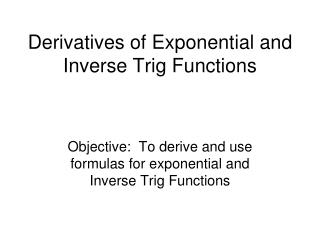DownloadDownload PresentationDerivatives of Exponential and Inverse Trig Functions

# Derivatives of Exponential and Inverse Trig Functions

Download Presentation## Derivatives of Exponential and Inverse Trig Functions

- - - - - - - - - - - - - - - - - - - - - - - - - - - E N D - - - - - - - - - - - - - - - - - - - - - - - - - - -
##### Presentation Transcript

1. Derivatives of Exponential and Inverse Trig Functions Objective: To derive and use formulas for exponential and Inverse Trig Functions

2. Derivatives of Exponential Functions • We will use our knowledge of logs to find the derivative of . We are looking for dy/dx.

3. Derivatives of Exponential Functions • We will use our knowledge of logs to find the derivative of . We are looking for dy/dx. • We know that is the same as

4. Derivatives of Exponential Functions • We will use our knowledge of logs to find the derivative of . We are looking for dy/dx. • We know that is the same as • We will take the derivative with respect to x and simplify.

5. Derivatives of Exponential Functions • We will use our knowledge of logs to find the derivative of . We are looking for dy/dx. • We know that is the same as • We will take the derivative with respect to x and simplify. • Remember that so

6. Derivatives of Exponential Functions • This formula, works with any base, so if the base is e, it becomes but remember , so

7. Derivatives of Exponential Functions • With the chain rule these formulas become:

8. Example 3 • Find the following derivatives:

9. Example 3 • Find the following derivatives:

10. Example 3 • Find the following derivatives:

11. Example 3 • Find the following derivatives:

12. Example 3 • Find the following derivatives:

13. Example 3 • Find the following derivatives:

14. Example 3 • Find the following derivatives:

15. Example 3 • Find the following derivatives:

16. Example 4 • Use logarithmic differentiation to find

17. Example 4 • Use logarithmic differentiation to find • Let

18. Example 4 • Use logarithmic differentiation to find • Let

19. Example 4 • Use logarithmic differentiation to find • Let

20. Example 4 • Use logarithmic differentiation to find

21. Example 4 • Use logarithmic differentiation to find

22. Derivatives of Inverse Trig Functions

23. Example 5 • Find dy/dx if:

24. Example 5 • Find dy/dx if:

25. Example 5 • Find dy/dx if:

26. Homework • Page 254 • 11-21 odd • 27, 33-41 odd